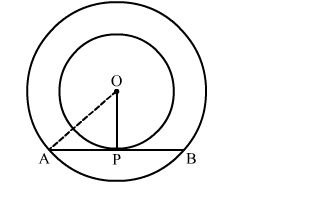# In the given figure, O is the centre of two concentric circles of radii 6 cm and 10 cm.`
Question:

In the given figure, O is the centre of two concentric circles of radii 6 cm and 10 cm. AB is a chord of outer circle which touches the inner circle. The length of AB is
(a) 8 cm
(b) 14 cm
(c) 16 cm

(d) $\sqrt{136} \mathrm{~cm}$Solution:

We know that the radius and tangent are perperpendular at their point of contact
In right  triangle AOP
AO2 = OP2 + PA2
⇒ 102 = 62 + PA2
⇒ PA2 = 64
⇒ PA = 8 cm
Since, the perpendicular drawn from the centre bisect the chord.
∴ PA = PB = 8 cm
Now, AB = AP + PB = 8 + 8 = 16 cm
Hence, the correct answer is option (c).